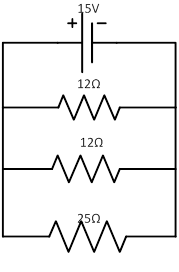### Sample ProblemWhat is the voltage of the 25 Ω resistor?

#### Solution

In a parallel circuit, VT = V1 = V2 = V3 = …

Therefore, the voltage of the 25 Ω resistor is equal to the total voltage, 15 V.